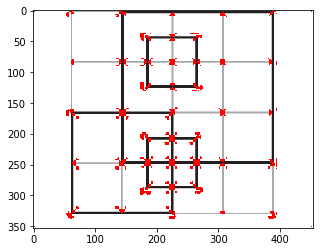# Harris Corner Detector implementation in python

The Harris corner detection algorithm also called the Harris & Stephens corner detector is one of the simplest corner detectors available. The idea is to locate interest points where the surrounding neighbourhood shows edges in more than one direction. The basic idea of algorithm is to find the difference in intensity for a displacement of (u,v) in all directions which is expressed as below: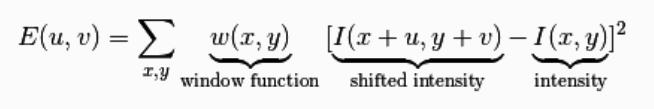Window function is either a rectangular window or a gaussian window which gives weights to pixels at (x,y). The above equation can be further approximated using Tayler expansion which gives us the final formula as: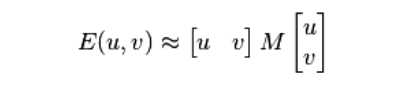where,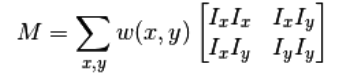Ix and Iy are image derivatives in x and y directions respectively. One can compute the derivative using the sobel kernel.

Then we finally find the Harris response R given by: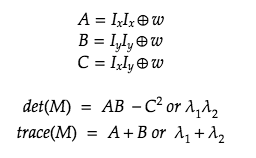where A, B and C are shifts of window defined by w. The lambdas are the Eigen values of M.

We find the corners using the value of R.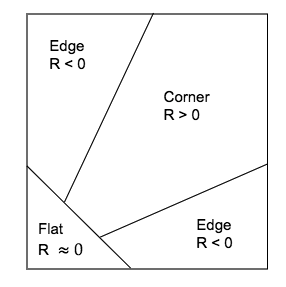#### Process of Harris Corner Detection Algorithm

1. Color image to Grayscale conversion
2. Spatial derivative calculation
3. Structure tensor setup
4. Harris response calculation
5. Find edges and corners using R

You can find the entire source code in this notebook. I am explaining the various parts of the same code here on, so its better you keep that open too for better understanding.

Lets take a small image and find edges and corners. For my sample implementation, I am taking the below image: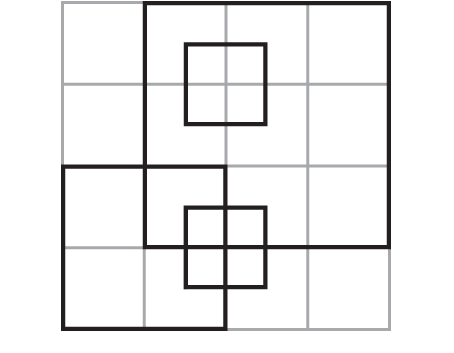### Step 1: Covert the image to Grayscale

```from skimage.io import imread
from skimage.color import rgb2gray

imggray = rgb2gray(img)```

output: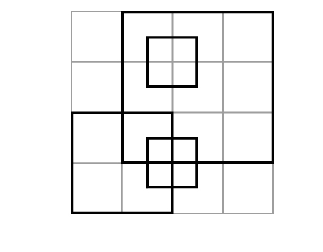You cannot see much difference but at the array level this is what I have:### Step 2: Spatial derivative calculation

```from scipy import signal as sig
import numpy as np

##Sobel operator kernels.
kernel_x = np.array([[-1, 0, 1],[-2, 0, 2],[-1, 0, 1]])
return sig.convolve2d(imggray, kernel_x, mode='same')
kernel_y = np.array([[1, 2, 1], [0, 0, 0], [-1, -2, -1]])
return sig.convolve2d(imggray, kernel_y, mode='same')

I am using the Sobel filter to find the derivatives. For the sake of simplicity, I am using the image as it is. The open-source implementations of the sobel derivative will convert the image to float before running convolution. You can check the source here.

### Step 3: Structure tensor setup

```Ixx = ndi.gaussian_filter(I_x**2, sigma=1)
Ixy = ndi.gaussian_filter(I_y*I_x, sigma=1)
Iyy = ndi.gaussian_filter(I_y**2, sigma=1)```

### Step 4: Harris response calculation

As described in the formulas above, we can easily calculate the response as shown in the code below.

```k = 0.05

# determinant
detA = Ixx * Iyy - Ixy ** 2
# trace
traceA = Ixx + Iyy

harris_response = detA - k * traceA ** 2```

We can find the Harris response by using the Summation as well. We first calculate the sum of squares of our gradient at each pixel by shifting a window over all the pixels in our image. The window is shifted according to the offset which you decided based on your image.

```for y in range(offset, height-offset):
for x in range(offset, width-offset):
Sxx = np.sum(Ixx[y-offset:y+1+offset, x-offset:x+1+offset])
Syy = np.sum(Iyy[y-offset:y+1+offset, x-offset:x+1+offset])
Sxy = np.sum(Ixy[y-offset:y+1+offset, x-offset:x+1+offset])```

Take a look at the below trace to get a rough idea of how the window shifting works. This is done for all Ixx, Iyy and Ixy.Using the sum of squares of each window we find the harris response R at each pixel.

```#Find determinant and trace, use to get corner response
det = (Sxx * Syy) - (Sxy**2)
trace = Sxx + Syy
r = det - k*(trace**2)

```

k is the sensitivity factor to separate corners from edges, typically a value close to zero, for my analysis I have taken k=0.04. Small values of k result in detection of sharp corners. The response R is an array of peak values of each row in the image. We can use these peak values to isolate corners and edges as I will describe in the next step.

### Step 5: Find edges and corners using R

1. Edge : r < 0
2. Corner : r > 0
3. Flat: r = 0
```img_copy_for_corners = np.copy(img)
img_copy_for_edges = np.copy(img)

for rowindex, response in enumerate(harris_response):
for colindex, r in enumerate(response):
if r > 0:
# this is a corner
img_copy_for_corners[rowindex, colindex] = [255,0,0]
elif r < 0:
# this is an edge
img_copy_for_edges[rowindex, colindex] = [0,255,0]```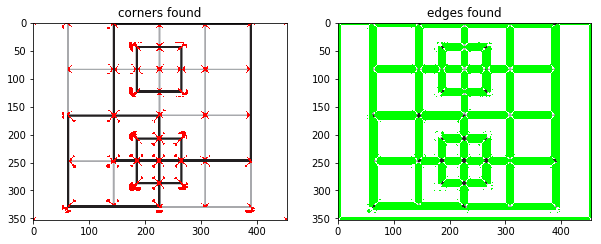You can see too make corners detected in the image, because the algorithm identifies the corner regions. To find the local maxima of the peaks we can use a peak finding algorithm provided in skimage.

```corners = corner_peaks(harris_response)
fig, ax = plt.subplots()
ax.imshow(img, interpolation='nearest', cmap=plt.cm.gray)
ax.plot(corners[:, 1], corners[:, 0], '.r', markersize=3)```

The output from the above code is as below: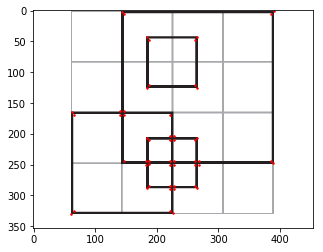The standard implementation provided in skimage or opencv used different variants of guassian filters and kernels to identify the corners so they may differ in their output. Below code is from skimage to detect corners. The output is quite close to what we have derived.

```#Harris corner detection using skimage library
from skimage.feature import corner_harris, corner_peaks
coords = corner_peaks(skimage_harris_response)

fig, ax = plt.subplots()
ax.imshow(img, interpolation='nearest', cmap=plt.cm.gray)
ax.plot(coords[:, 1], coords[:, 0], '.r', markersize=3)```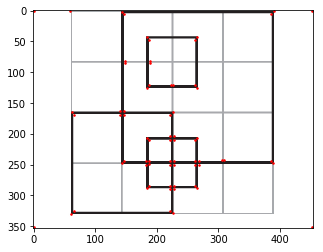References:

0 0 vote
Article Rating
Subscribe
Notify ofInline FeedbacksAjith
11 months ago

Could you post the complete programAjith
11 months ago

How to print the corner points? .I am not able to get the corner points on which red points are drawnAline
2 months ago

Hi, I reproduced your harris algorithm, but I couldn’t detect the corners.
I didn’t use skimage, I just need to use the harris.

6
0